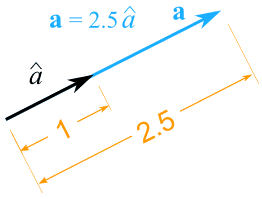# Unit, Free, Forced and Fixed Vector

UNIT VECTOR :A unit vector is defined as a vector in any specified direction whose magnitude is unity i.e. 1. A unit vector only specifies the direction of a given vector. ”A free vector : is one whose action is not confined to or associated with a unique line in space. For example if a body is in translational motion, velocity of any point in the body may be taken as a vector and this vector will describe equally well the velocity of every point in the body. Hence, we may represent the velocity of such a body by a free vector. In statics, couple moment is a free vector.

Sliding Vector: A vector that can be applied at any point on a body so long as it is along its original line of action and doesn’t change its affect on the body as a whole. Maintains direction & magnitude.
Example: On the front of this card you will see an airplane. In figure 1 a tailwind of 350 N is applied to the rear of the plane, pushing it forward. This vector is “slid” forward along its line of action (thin black line) and in figure 2 represents the thrust provided by the prop. which happens to also be 350 N. Since the magnitude and direction are the same along with the line of action, this is a sliding vector.

Fixed Vector: A vector that has a unique point of application and can be treated as a sliding vector by being slid along its line of action in order to examine the external effects it has on a body (How it affects the body as a whole).
Example: The submarine shown on the front has 2 vectors acting on it: an upthrust buoyancy vector and a weight vector. These two are “slid” along their line of action to examine the external effects they have on the submarine. NOTE: The internal effects cannot be examined using a fixed vector.

### Feedback is important to us.#### Satya Prakash

Mr. Satya Prakash is a research scholar.

error: Content is protected !!